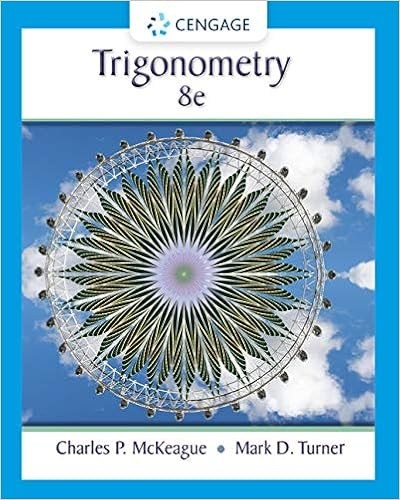# A roller coaster starts from rest at point a if you

• Notes
• 10
• 100% (1) 1 out of 1 people found this document helpful

This preview shows page 9 - 10 out of 10 pages.

##### We have textbook solutions for you!
The document you are viewing contains questions related to this textbook.The document you are viewing contains questions related to this textbook.
Chapter 3 / Exercise 57
Trigonometry
McKeague/TurnerExpert Verified
21. A roller coaster starts from rest at point A. If you ignore friction and take the zero of potential energy to be at C, A) the kinetic energy of the coaster at D will be equal to its potential energy at A. B) the kinetic energy of the coaster at E will be equal to its potential energy at C. C) the kinetic energy of the coaster at A will be equal to its potential energy at B. D) the kinetic energy of the coaster at B will be equal to its potential energy at C. E) the kinetic energy of the coaster at C will be equal to its potential energy at A.
22. Assuming the incline to be frictionless and the zero of gravitational potential energy to be at the elevation of the horizontal line,
.
.
1
##### We have textbook solutions for you!
The document you are viewing contains questions related to this textbook.The document you are viewing contains questions related to this textbook.
Chapter 3 / Exercise 57
Trigonometry
McKeague/TurnerExpert Verified
Page 10 23. The surface shown in the figure is frictionless. The block is released from rest. What will be the maximum compression of the spring? (Provide three significant digits in the final answer.)
•••